Get instant live expert help with Excel or Google Sheets“My Excelchat expert helped me in less than 20 minutes, saving me what would have been 5 hours of work!”

#### Post your problem and you’ll get expert help in seconds.

Your message must be at least 40 characters
Our professional experts are available now. Your privacy is guaranteed.

# The Procedure for Calculating a Percentage in a Pivot Table

Are you struggling with calculating a percentage in a pivot table? Pivot tables in Excel are excellent tools for analyzing data. They help you to aggregate, summarize, finding insights and presenting a large amount of data in just a few clicks, including calculating a percentage from given data.

You can create and modify pivot tables very quickly. You can create calculated fields in a pivot table that help expand your analysis with more data. Though it has some limitations, calculated fields are a great way to find new insights, such as percentages, from pivot tables.

## Calculating percentage in the pivot table

In this example, you have the beverage sales data of eleven items for the 3rd quarter of the year.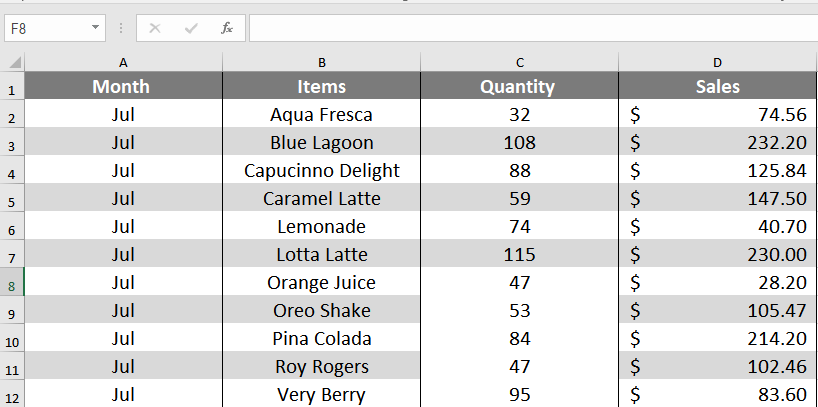### Create and format your pivot table

To create the Pivot Table and apply conditional formatting, you need to perform the following steps:

1. Click anywhere in the data.
2. Go to Insert > Recommended PivotTables. Scroll down and select the one that says Sum of Sales by Items and Month.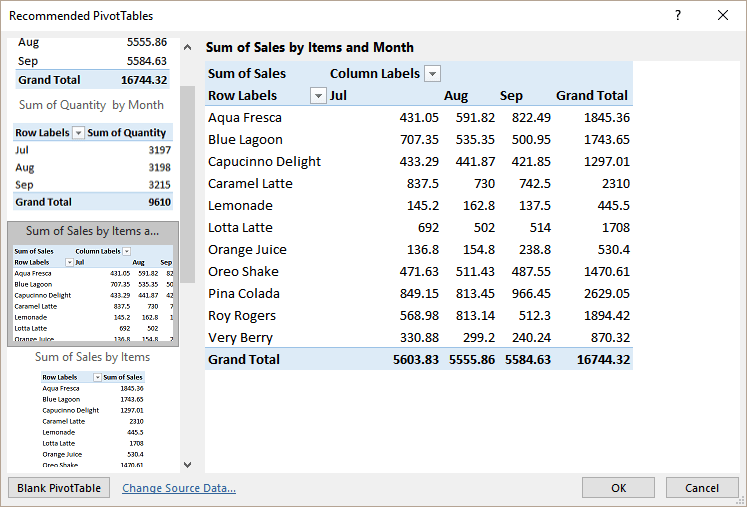3. Click OK.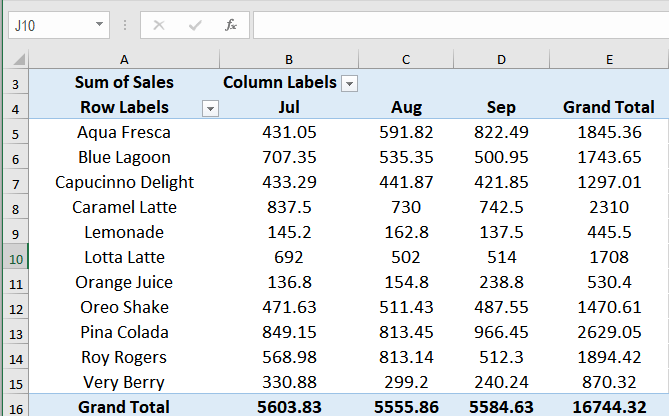You will have the pivot table with the Sales for the Items for each Month.

To calculate % of Sales for each month, you need to do the following: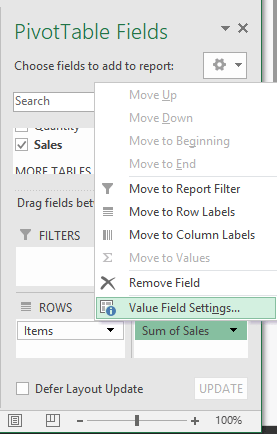1. Click on pivot builder the entry Sum of Sales and select Value Field Settings.
2. In the Value Field Settings window, on the Show Values As tab, choose % of Column Total.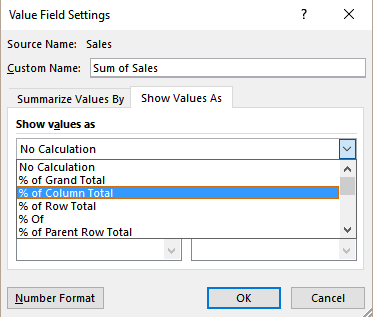3. Click OK.

This would show the sales for each item as the percentage of total monthly sales.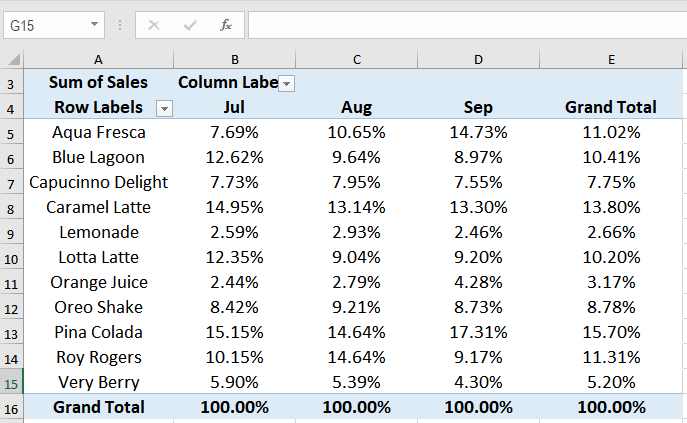### Create the calculated field in the pivot table

A calculated field is a column generated by the data in the pivot table. For this example, we will use the sales and profit data for the eleven items during the 4th quarter of the year.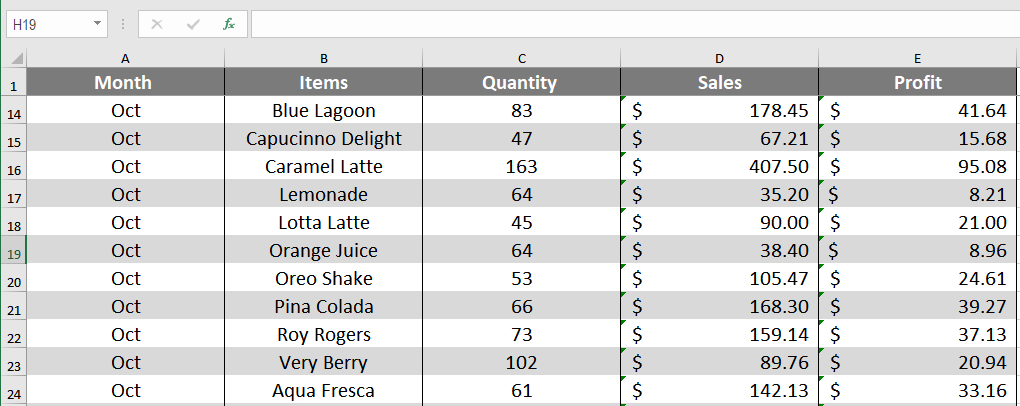From this, we have the pivot table Sum of Sales and Profits for the Items.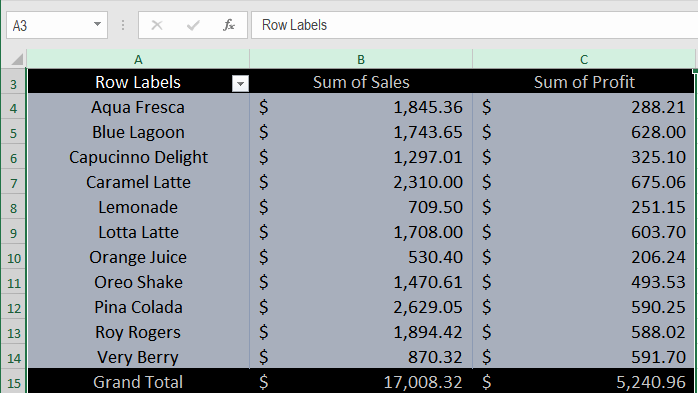To add the profit margin for each item:

1. Click on any cell in the Pivot Table.
2. Go to (Pivot Table Tools) Analyze > Fields, Items, & Sets > Calculated Field.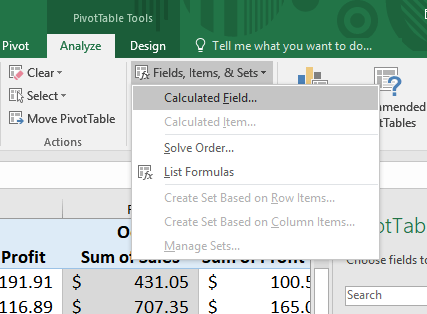3. In the Insert Calculated Field dialog box,
• Assign a name in the Name field.
• In the Formula field, insert the formula `=Profit/Sales` by clicking on the Insert Field button from the Fields box.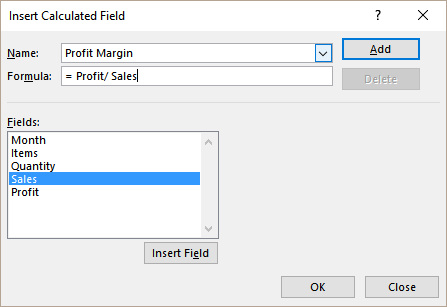4. Click ADD and then OK.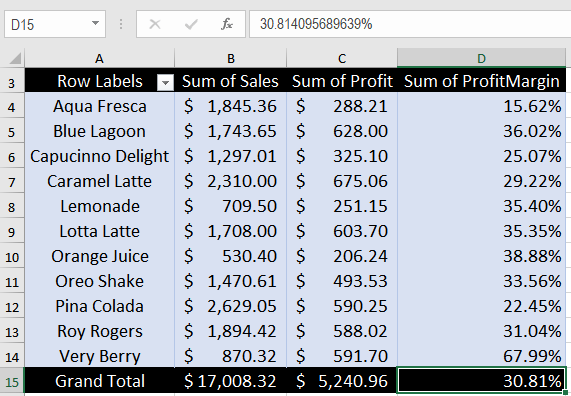### Limitation of the calculated fields in the pivot table when calculating a percentage

Calculated fields in pivot table have some limitations. A calculated field is always performed against the SUM of the data. This limits you from using a lot of functions. Furthermore, you cannot use any functions that require cell references or defined names as an argument. This rules out functions like COUNT, AVERAGE, IF, AND, NOT, and OR.

In the following example, you will use the Clayton High School Track and Field club’s event record for the past six months. It includes the EventNames and Win records. You will calculate the count of wins as a percentage for the count of athletes based on the events.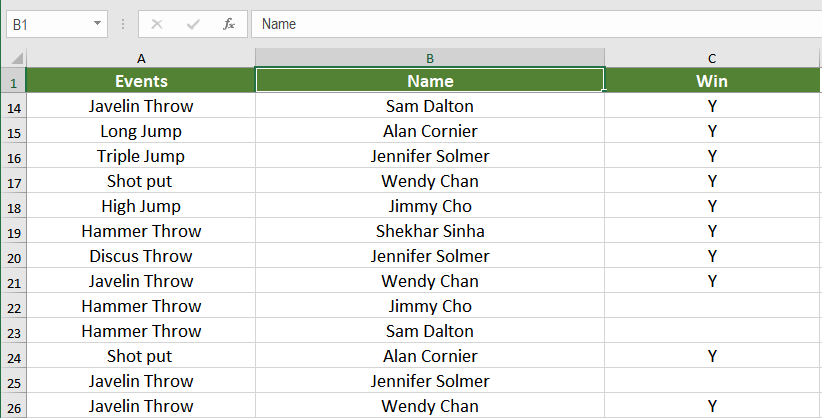From this, we have the pivot table Count of Name and Count of Win.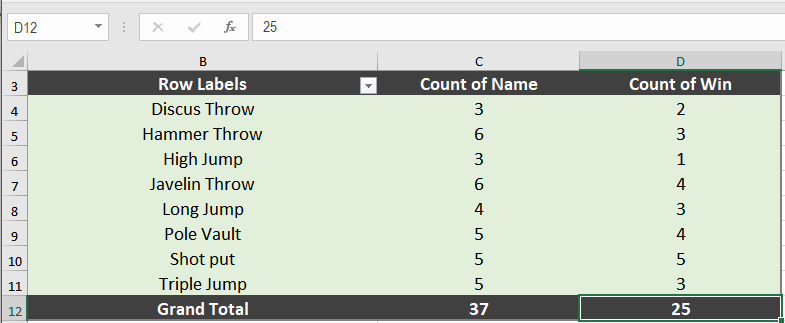To find the count of wins as a percentage for the count of athletes based on events at first, you will try with a calculated field. Add a calculated field like the previous section named Win Percentage and having the formula =Win / Name.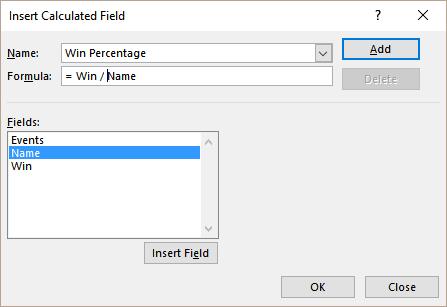As calculated field only performs calculations against the SUM of data, we get a #DIV/0 error.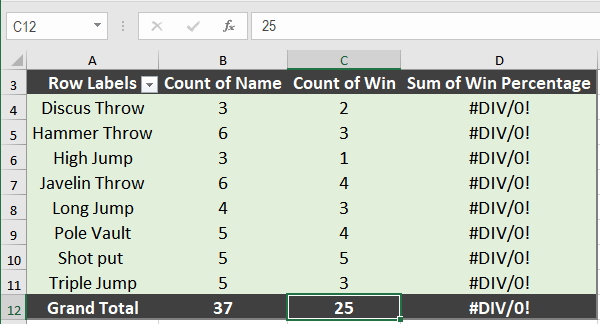To overcome this issue, you need to follow the next steps:

1. Make sure you have Power Pivot enabled in File > Options > Add-Ins.
2. Click COM Add-ins in the Manage box > Click Go.
3. Check the box for Microsoft Office Power Pivot > click OK. Select the Power Pivot add-in for Excel if you have other versions of power pivot installed.From the Events_Record worksheet, go to Power Pivot > Manage.

You will use Data analysis expression (DAX) to create calculated fields in Power Pivot. DAX is used to add calculations. A measure is a formula that is created specifically for use in a pivot table that uses data in the Power Pivot. You will use the measure in the Values area of the pivot table.

1. In the Power Pivot window, Click Home> View> Calculation Area.2. Click on an empty cell in the Calculation Area.
3. In the formula bar, at the top of the table, enter the formula,`% of wins := DIVIDE(CALCULATE(COUNTA([Win]),FILTER(Table1,Table1[Win]="Y")),COUNTA([Name]),0)`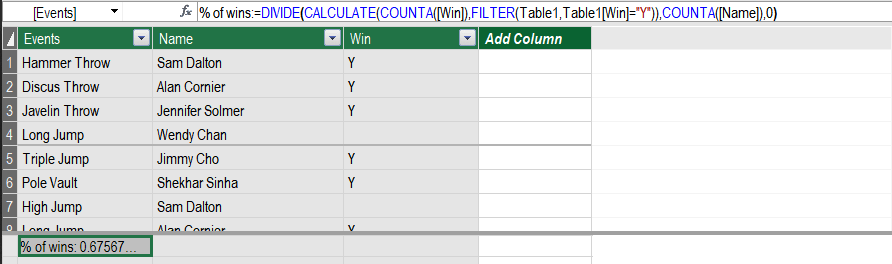4. Press Enter to accept the formula.5. Click anywhere in the Power Pivot data. Go to Home > PivotTable.
6. In the Create PivotTable dialogue box, check New Worksheet.
7. In the resulting pivot table worksheet, expand Table1 in the PivotTable Fields Menu on the right. Drag Events to the Row field. NameWinand fx % of wins to the Values field.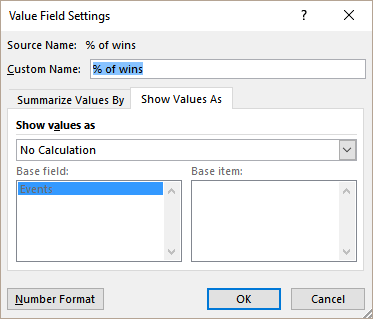8. Right-click anywhere in the % of wins column in the pivot table. Select Value Field Settings > Show Values As > Number Format > Percentage. Click OK twice.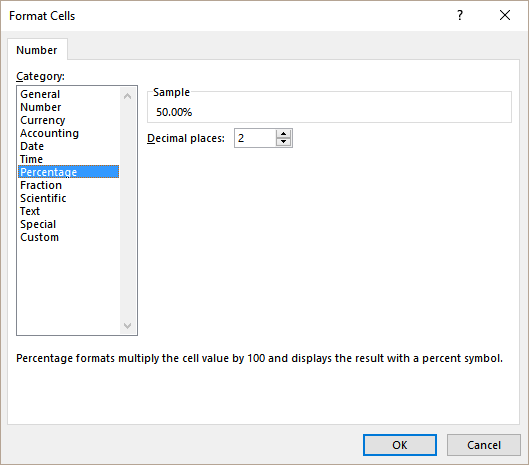This will show the count of wins as a percentage for the count of athletes based on the events.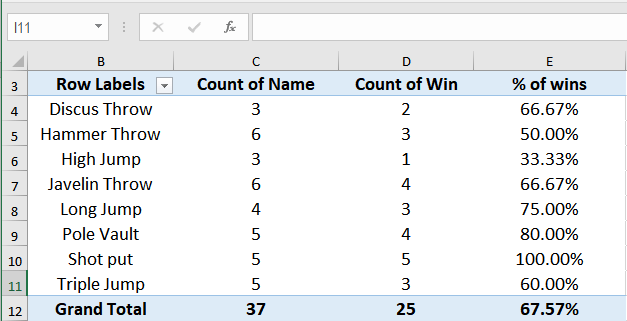Pivot tables are a great way to summarize and aggregate data to model and present it. You can now visualize and report data in the blink of an eye. Dashboards and other features have made gaining insights very simple using pivot tables. Calculated fields in the pivot table is a great way to create formulas to add a sum of columns.

There are many powerful features of Pivot Tables, Power Pivot, and Data analysis expression (DAX) that could help you gain insights into your data. If you want to save hours of researching and frustration and get to the solution quickly, try our Excel Live Chat service! Our Excel experts are available 24/7 to answer any Excel question you have on the spot. The first question is free.

### Did this post not answer your question? Get a solution from connecting with the expert.Another blog reader asked this question today on Excelchat:
Related blogs
Solution examplesI need a formula that take a total amount, which is in cell c37, then times it by .015 and then adds 15. Then, add d2 and a5 and if the results show 400 or more, it will add .10 per every number 400 and above. Once it has done all that, I need it to times 1.2 for the VAT.
Solved by M. S. in 34 minsI need to copy a value from one column to another when text in a another column says "win"
Solved by E. E. in 20 minsHi, i want to create a formula which allows a different calculation based on what volume is inputted. for example if a customer buys 500 units than my formula will multiply 90 per unit, however if i input 1300 units then my formula will multiply by 80 per unit, again if i input 2600, my formula will multiply by 57.14. My price-breaks are >500-<1100= multiply by 90 & >1300 - <1900 = multiply by 80 & >2600-<3500 = multiply by 57.14. I have managed to achieve this with IF for just 1 SUM but cannot work out how to get all three calculations working in 1 formula. please help :)
Solved by B. J. in 15 minsi need a formula to fill a cell with a number value based on entries in two adjacent cells. for example, if A1='F' and B1='3', then I would like C1 to autofill with '18'
Solved by A. H. in 20 minsindexing multiple rows of data from criteria in a column (need to be able to bring up multiple job specifics [each job has its own row] by searching in the "due date" column for jobs due between start date and end date
Solved by O. S. in 55 mins## Subscribe to Excelchat.coAnother blog reader asked this question today on Excelchat: